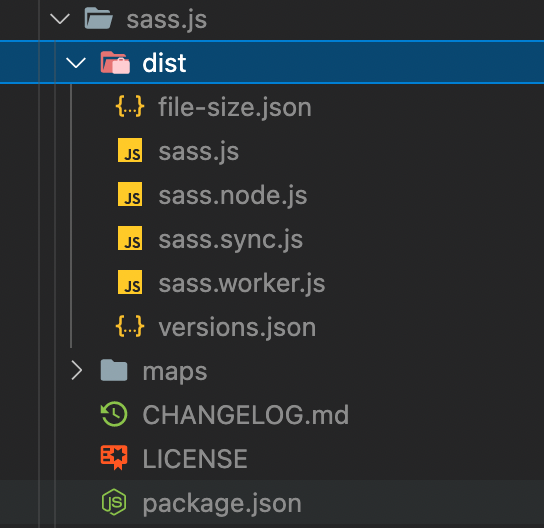Published on

# 实现一个 Code Pen：（四）浏览器编译代码

Authors
•作者
狂奔滴小马

## Iframe 实时运行

``````import React, { useState, useEffect } from 'react'
import Editor from './Editor'
import { useLocalStorage } from 'react-use'

function App() {
const [html, setHtml] = useLocalStorage('html', '')
const [css, setCss] = useLocalStorage('css', '')
const [js, setJs] = useLocalStorage('js', '')
const [srcDoc, setSrcDoc] = useState('')

useEffect(() => {
const timeout = setTimeout(() => {
setSrcDoc(`
<html>
<style>\${css}</style>
<body>\${html}</body>
<script>\${js}</script>
</html>
`)
}, 800)

return () => clearTimeout(timeout)
}, [html, css, js])

return (
<>
<div className="pane">
<Editor language="html" value={html} onChange={setHtml} />
<Editor language="css" value={css} onChange={setCss} />
<Editor language="javascript" value={js} onChange={setJs} />
</div>
<div className="pane">
<iframe
srcDoc={srcDoc}
title="output"
sandbox="allow-scripts"
frameBorder="0"
width="100%"
height="100%"
/>
</div>
</>
)
}

export default App
``````

## JS 编译

``````<script src="https://unpkg.com/@babel/standalone/babel.min.js"></script>
<script type="text/babel" data-presets="typescript">
const x: number = 0
console.log(x)
</script>
``````

``````import * as Babel from '@babel/standalone'

function compileJs(code) {
const res = Babel.transform(code, {
presets: ['react'],
})
return res.code
}
``````

## 编译 typescript

``````function compileTs(code) {
const res = Babel.transform(code, {
presets: ['typescript'],
filename: 'index.ts',
})
return res.code
}
``````

## Less 编译

1. 在 Node.js 环境中使用 Less
``````npm install -g less
lessc styles.less styles.css
``````
1. 在浏览器环境中使用 Less
``````<link rel="stylesheet/less" type="text/css" href="styles.less" />
<script src="//cdnjs.cloudflare.com/ajax/libs/less.js/3.11.1/less.min.js"></script>
``````
1. 我们的需求也是在浏览器中执行，但我们可以将编译的逻辑放在 web worker 中
``````import Less from 'less/lib/less'
const less = Less()

async function compileLess(code) {
return await less.render(code).then((res) => res.css)
}
``````

## Scss 编译

scss 编译我选择的是 sass.js

``````npm install -g sass.js
````````````import Sass from 'sass.js/dist/sass'
Sass.setWorkerUrl('/vendor/sass.worker.js')

function compileScss(code) {
const sass = new Sass()
return new Promise((resolve, reject) => {
sass.compile(code, (result) => {
if (result.status === 0) return resolve(result.text)
reject(new Error(result.formatted))
})
})
}
``````

## 小结